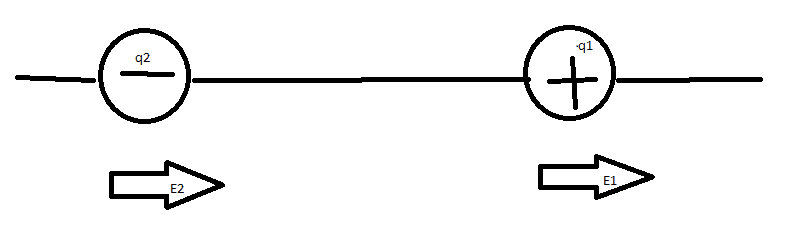# Position of two point charges

## Homework Statement

"Can you arrange the two point charges q1 =−2.0×10−6 C and q2 =4.0×10−6 C along the x-axis so that E =0 at the origin?"

## Homework Equations

E = K (|q|/r2)

K = Coloumb Constant

## The Attempt at a Solution

Since one particle is negatively charged and the other is positively charged, they'll both be pointing their fields down the same direction, just at different strengths. The distance between the two charges will be named with an r, and the distance between q1 to 0 will be x (meaning q2 distance to 0 is x+r)

0 = Eox = E2x + E1x
0 = Eox = K (|q2|/(x+r)2) + K (|q1|/x2)
0 = Eox = K (|q2|/(x+r)2 + |q1|/x2)
0 = |q2|/(x2(x+r)2) + |q1|/(x2(x+r)2
0 = |q2|/(x2 + |q1| (x+r)2)/(x2(x+r)2

0 = |q2|x2 + |q1| (x+r)2

Before I go any further, this doesn't look correct. Am I on the right path?

## Answers and Replies

haruspex
Science Advisor
Homework Helper
Gold Member
2020 Award
Since one particle is negatively charged and the other is positively charged, they'll both be pointing their fields down the same direction, just at different strengths.
Not sure what you mean. It sounds wrong.
0 = Eox = K (|q2|/(x+r)2) + K (|q1|/x2)
Why the absolute value signs?

You should not have absolute values on the charges. The sign of the charges will change the answer. You are on the right path defining the variables ##x## and ##r## to describe the displacement. There will be multiple values of ##x## and ##r## which give a zero field at the origin, but there will be two ratios of ##x## and ##r## which do this. I would suggest you define a new variable ##y=r/x## and solve for this ratio.

gneill
Mentor
It's often easier to start with a sketch. Place the two charges on a line (don't locate the origin yet) and sketch in approximated vectors for the fields due to each charge in the three distinct regions along the line. You should be able to use a bit of thought to find the only region where the vectors can sum to zero.

While it's possible to solve the problem purely algebraically, the gymnastics you have to perform to take into account the vector directions for every position can be tiresome. Draw a picture and find your target region by inspection.This is what I sketched earlier. So E2 and E1 will both be in the same direction.

The 'absolute value' came from my notes, and it's supposed to just represent the magnitude of the charge.

#### Attachments

haruspex
Science Advisor
Homework Helper
Gold Member
2020 Award
The 'absolute value' came from my notes, and it's supposed to just represent the magnitude of the charge.
It does, but the formula for the field at a given distance uses the signed charge, not the magnitude.
So E2 and E1 will both be in the same direction.
Your arrows make no sense.
Start with just a positive charge. In what directions are the field lines?

Okay, I asked my professor. I believe I see where my error is now.

Since the fields need to equate to 0, they need to cancel each other out.

q1/r12 = q2/r22 (r2 = x+r1)
q1r22=q2r12
q1/q2 = r12 / r22
q1/q2 = r12 / r22
√q1/q2 =√ r12 / r22
√q1/q2 * r2 = r1

And then just plug in r2 = x+r1 and find the ratio from there.

Now does that look good?

haruspex
Science Advisor
Homework Helper
Gold Member
2020 Award
Okay, I asked my professor. I believe I see where my error is now.

Since the fields need to equate to 0, they need to cancel each other out.

q1/r12 = q2/r22 (r2 = x+r1)
q1r22=q2r12
q1/q2 = r12 / r22
q1/q2 = r12 / r22
√q1/q2 =√ r12 / r22
√q1/q2 * r2 = r1

And then just plug in r2 = x+r1 and find the ratio from there.

Now does that look good?
Yes, but you should use parentheses. E.g. √q1/q2 * r2 = r1 taken literally means (√q1)/q2 * r2 = r1, but you obviously mean √(q1/q2) * r2 = r1.
Also, I'm not sure you understand field directions.
What is the direction of the net field:
- left of the origin
- between the origin and q1
- between q1 and q2
- right of q2?

That "A" Is supposed to be a square root. I'll use parenthesis next time, apologies.

Also, I'm not sure you understand field directions.
What is the direction of the net field:
- left of the origin
- between the origin and q1
- between q1 and q2
- right of q2?

Wouldn't I need to have a value for X first, where that can't be determined directly (only have the ratio between q1's distance to 0 compared to q2's distance to 0)?

Anyway, I'll take a stab at it.

- down along the -x axis
- toward q1
- up the +x axis
- Up the +x axis

haruspex
Science Advisor
Homework Helper
Gold Member
2020 Award
down along the -x axis
Right. At great distance from the two charges, their separation ceases to matter. The stronger charge wins.
Since that is the positive charge, the field is away from them.
toward q1
Right. The fields cancel at the origin, and any nearer to q1 means q1 will win.
up the +x axis
No, between q1 and q2 the two fields act in the same direction, towards q1, away from q2.
Up the +x axis
Right.